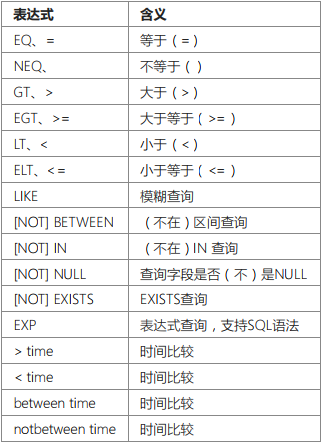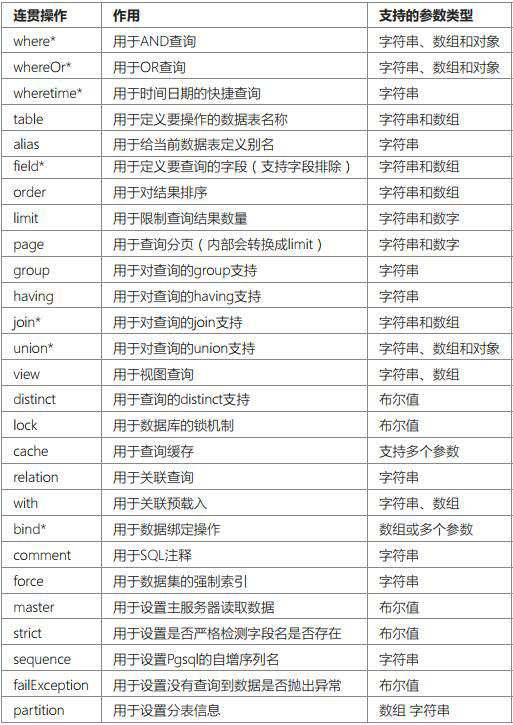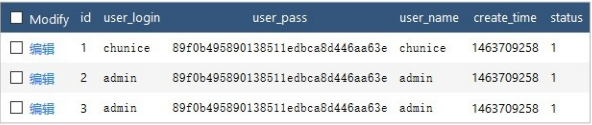# thinkPHP查询数据常用的3种方法 : 查询方法、查询语法、链式操作

where 方法

```Db::table('think_user')
->where('name','like','%thinkphp')
->where('status',1)
->find();```

```Db::table('think_user')
->where('name&title','like','%thinkphp')
->find();```

whereOr 方法

```Db::table('think_user')
->where('name','like','%thinkphp')
->whereOr('title','like','%thinkphp')
->find();```

```Db::table('think_user')
->where('name|title','like','%thinkphp')
->find();```

where方法和whereOr方法在复杂的查询条件中经常需要配合一起混合使用，下面举个例子：

```\$result = Db::table('think_user')->where(function (\$query) {
\$query->where('id', 1)->whereor('id', 2);
})->whereOr(function (\$query) {
\$query->where('name', 'like', 'think')->whereOr('name', 'like', 'thinkphp');
})->select();```

`SELECT * FROM `think_user` WHERE ( `id` = 1 OR `id` = 2 ) OR ( `name` LIKE 'think' OR `name` LIKE 'thinkphp' )`

getTableInfo 方法

```// 获取`think_user`表所有信息
Db::getTableInfo('think_user');
// 获取`think_user`表所有字段
Db::getTableInfo('think_user', 'fields');
// 获取`think_user`表所有字段的类型
Db::getTableInfo('think_user', 'type');
// 获取`think_user`表的主键
Db::getTableInfo('think_user', 'pk');```

```where('字段名','表达式','查询条件');
whereOr('字段名','表达式','查询条件');```EQ ：等于（=）

```where('id','eq',100);
where('id','=',100);```

`where('id',100);`

NEQ：不等于（）

```where('id','neq',100);
where('id','<>',100);```

GET：大于等于（>=）

```where('id','egt',100);
where('id','>=',100);```

LT：小于（<）

```where('id','lt',100);
where('id','<',100);```

ELT：小于等于（<=）

```where('id','elt',100);
where('id','<=',100);```

[NOT] LIKE： 同sql的LIKE

`where('name','like','thinkphp%')`

V5.0.5+ 版本开始，like查询支持使用数组

`where('name','like',['%think','php%'],'OR');`

[NOT] BETWEEN： 同sql的[not] between

```where('id','between','1,8');
where('id','between',[1,8]);
## 两条语句等效```

[NOT] IN：同sql的[not] in

```where('id','not in','1,5,8');
where('id','not in',[1,5,8]);
## 两条语句等效```

[NOT] NULL：查询字段是否（不）是`Null`

```where('name', null);
where('title','null');
where('name','not null');```

```where('title','=', 'null');
where('name','=', 'not null');```

EXP：表达式

`where('id','in','1,3,8');`

`where('id','exp',' IN (1,3,8) ');`

`exp`查询的条件不会被当成字符串，所以后面的查询条件可以使用任何SQL支持的语法，包括使用函数和字段名称。

```Db::table('think_user')
->where('status',1)
->order('create_time')
->limit(10)
->select();```

```Db::table('think_user')
->order('create_time')
->limit(10)
->where('status',1)
->select();```

```Db::table('think_user')
->where('id',1)
->field('id,name,email')
->find();
Db::table('think_user')
->where('status',1)
->where('id',1)
->delete();```where

where方法的用法是ThinkPHP查询语言的精髓，也是ThinkPHP ORM的重要组成部分和亮点所在，可以完成包括普通查询、表达式查询、快捷查询、区间查询、组合查询在内的查询操作。where方法的参数支持字符串和数组，虽然也可以使用对象但并不建议。

```Db::table('think_user')
->where('id','>',1)
->where('name','thinkphp')
->select();```

```\$map['name'] = 'thinkphp';
\$map['status'] = 1;
// 把查询条件传入查询方法
Db::table('think_user')->where(\$map)->select();
// 助手函数
db('user')->where(\$map)->select();```

`SELECT * FROM think_user WHERE `name`='thinkphp' AND status=1`

```\$map['id'] = ['>',1];
\$map['mail'] = ['like','%thinkphp@qq.com%'];
Db::table('think_user')->where(\$map)->select();```

`Db::table('think_user')->where('type=1 AND status=1')->select();`

`SELECT * FROM think_user WHERE type=1 AND status=1`

`Db::table('think_user')->where("id=:id and username=:name")->bind(['id'=>[1,\PDO::PARAM_INT],'name'=>'thinkphp'])->select();`

table

table方法主要用于指定操作的数据表。

1. 切换操作的数据表；

2. 对多表进行操作；

`Db::table('think_user')->where('status>1')->select();`

`Db::table('db_name.think_user')->where('status>1')->select();`

table方法指定的数据表需要完整的表名，但可以采用下面的方式简化数据表前缀的传入，例如：

`Db::table('__USER__')->where('status>1')->select();`

```Db::field('user.name,role.title')
->table('think_user user,think_role role')
->limit(10)->select();```

```Db::field('user.name,role.title')
->table(['think_user'=>'user','think_role'=>'role'])
->limit(10)->select();```

alias

alias用于设置当前数据表的别名，便于使用其他的连贯操作例如join方法等。

`Db::table('think_user')->alias('a')->join('__DEPT__ b ','b.user_id= a.id')->select();`

`SELECT * FROM think_user a INNER JOIN think_dept b ON b.user_id= a.id`

v5.0.2+ 版本开始，可以传入数组批量设置数据表以及别名，例如：

`Db::table('think_user')->alias(['think_user'=>'user','think_dept'=>'dept'])->join('think_dept','dept.user_id= user.id')->select();`

`SELECT * FROM think_user user INNER JOIN think_dept dept ON dept.user_id= user.id`

field

field方法属于模型的连贯操作方法之一，主要目的是标识要返回或者操作的字段，可以用于查询和写入操作。

`Db::table('think_user')->field('id,title,content')->select();`

`SELECT id,title,content FROM table`

`Db::table('think_user')->field('id,nickname as name')->select();`

`SELECT id,nickname as name FROM table`

`Db::table('think_user')->field('id,SUM(score)')->select();`

`SELECT id,SUM(score) FROM table`

field方法的参数可以支持数组，例如：

`Db::table('think_user')->field(['id','title','content'])->select();`

`Db::table('think_user')->field(['id','nickname'=>'name'])->select();`

`SELECT id,nickname as name FROM table`

`Db::table('think_user')->field(['id','concat(name,"-",id)'=>'truename','LEFT(title,7)'=>'sub_title'])->select();`

`SELECT id,concat(name,'-',id) as truename,LEFT(title,7) as sub_title FROM table`

```Db::table('think_user')->select();
Db::table('think_user')->field('*')->select();```

`SELECT * FROM table`

`Db::table('think_user')->field(true)->select();`

field(true) 的用法会显式的获取数据表的所有字段列表，哪怕你的数据表有100个字段。

`Db::table('think_user')->field('content',true)->select();`

```Db::table('think_user')->field('user_id,content',true)->select();
//或者用
Db::table('think_user')->field(['user_id','content'],true)->select();```

`Db::table('think_user')->field('title,email,content')->insert(\$data);`

order

order方法属于模型的连贯操作方法之一，用于对操作的结果排序。

`Db::table('think_user')->where('status=1')->order('id desc')->limit(5)->select();`

`Db::table('think_user')->where('status=1')->order('id desc,status')->limit(5)->select();`

`Db::table('think_user')->where('status=1')->order(['order','id'=>'desc'])->limit(5)->select();`

limit

limit方法也是模型类的连贯操作方法之一，主要用于指定查询和操作的数量，特别在分页查询的时候使用较多。

ThinkPHP的limit方法可以兼容所有的数据库驱动类的。

```Db::table('think_user')
->where('status=1')
->field('id,name')
->limit(10)
->select();```

limit方法也可以用于写操作，例如更新满足要求的3条数据：

```Db::table('think_user')
->where('score=100')
->limit(3)
->update(['level'=>'A']);```

`Db::table('think_article')->limit('10,25')->select();`

`Db::table('think_article')->limit(10,25)->select();`

page

page方法也是模型的连贯操作方法之一，是完全为分页查询而诞生的一个人性化操作方法。

```// 查询第一页数据
Db::table('think_article')->limit('0,10')->select();
// 查询第二页数据
Db::table('think_article')->limit('10,10')->select();```

```// 查询第一页数据
Db::table('think_article')->page('1,10')->select();
// 查询第二页数据
Db::table('think_article')->page('2,10')->select();```

```Db::table('think_article')->page(1,10)->select();
// 和下面的用法等效
Db::table('think_article')->page('1,10')->select();```

page方法还可以和limit方法配合使用，例如：

`Db::table('think_article')->limit(25)->page(3)->select();`

`Db::table('think_article')->page('3,25')->select();`

group

GROUP方法也是连贯操作方法之一，通常用于结合合计函数，根据一个或多个列对结果集进行分组。

group方法只有一个参数，并且只能使用字符串。

```Db::table('think_user')
->group('user_id')
->select();```

`SELECT user_id,username,max(score) FROM think_score GROUP BY user_id`

```Db::table('think_user')
->group('user_id,test_time')
->select();```

`SELECT user_id,test_time,username,max(score) FROM think_score GROUP BY user_id,test_time`

having

HAVING方法也是连贯操作之一，用于配合group方法完成从分组的结果中筛选（通常是聚合条件）数据。

having方法只有一个参数，并且只能使用字符串，例如：

```Db::table('think_user')
->group('user_id')
->having('count(test_time)>3')
->select();```

`SELECT username,max(score) FROM think_score GROUP BY user_id HAVING count(test_time)>3`

join

join通常有下面几种类型，不同类型的join操作会影响返回的数据结果。

• INNER JOIN: 等同于 JOIN（默认的JOIN类型）,如果表中有至少一个匹配，则返回行
• LEFT JOIN: 即使右表中没有匹配，也从左表返回所有的行
• RIGHT JOIN: 即使左表中没有匹配，也从右表返回所有的行
• FULL JOIN: 只要其中一个表中存在匹配，就返回行

`object join ( mixed join [, mixed \$condition = null [, string \$type = 'INNER']] )`

JOIN方法也是连贯操作方法之一，用于根据两个或多个表中的列之间的关系，从这些表中查询数据。

join

• 写法1：[ ‘完整表名或者子查询’=>’别名’ ]
• 写法2：’完整表名 别名’
• 写法3：’不带数据表前缀的表名’

condition

type

```Db::table('think_artist')
->alias('a')
->join('think_work w','a.id = w.artist_id')
->join('think_card c','a.card_id = c.id')
->select();
Db::table('think_artist')
->alias('a')
->join('__WORK__ w','a.id = w.artist_id')
->join('__CARD__ c','a.card_id = c.id')
->select();
\$join = [
['think_work w','a.id=w.artist_id'],
['think_card c','a.card_id=c.id'],
];
Db::table('think_user')->alias('a')->join(\$join)->select();```

`Db::table('think_user')->join('__WORK__','__ARTIST__.id = __WORK__.artist_id')->select();`

`Db::table('think_user')->alias('a')->join('word w','a.id = w.artist_id','RIGHT')->select();`

```\$subsql = Db::table('think_work')->where(['status'=>1])->field('artist_id,count(id) count')->group('artist_id')->buildSql();
Db::table('think_user')->alias('a')->join([\$subsql=> 'w'], 'a.artist_id = w.artist_id')->select();```

union

UNION操作用于合并两个或多个 SELECT语句的结果集。

```Db::field('name')
->table('think_user_0')
->union('SELECT name FROM think_user_1')
->union('SELECT name FROM think_user_2')
->select();```

```Db::field('name')
->table('think_user_0')
->union(function(\$query){
\$query->field('name')->table('think_user_1');
})
->union(function(\$query){
\$query->field('name')->table('think_user_2');
})
->select();```

```Db::field('name')
->table('think_user_0')
->union(['SELECT name FROM think_user_1','SELECT name FROM think_user_2'])
->select();```

```Db::field('name')
->table('think_user_0')
->union('SELECT name FROM think_user_1',true)
->union('SELECT name FROM think_user_2',true)
->select();```

```Db::field('name')
->table('think_user_0')
->union(['SELECT name FROM think_user_1','SELECT name FROM think_user_2'],true)
->select();```

distinct

DISTINCT 方法用于返回唯一不同的值 。`Db::table('think_user')->distinct(true)->field('user_login')->select();`

`SELECT DISTINCT user_login FROM think_user`

```array(2) {
 => array(1) {
}
 => array(1) {
}
}```

distinct方法的参数是一个布尔值。

lock

Lock方法是用于数据库的锁机制，如果在查询或者执行操作的时候使用：

`lock(true);`

cache

cache方法用于查询缓存操作，也是连贯操作方法之一。 cache可以用于 select 、 find 、 value 和 column 方法，以及其衍生方法，使用 cache 方法后，在缓存有效期之内不会再次进行数据库查询操作，而是直接获取缓存中的数据，关于数据缓存的类型和设置可以参考缓存部分。

`Db::table('think_user')->where('id=5')->cache(true)->find();`

```Db::table('think_user')->cache(true,60)->find();
// 或者使用下面的方式 是等效的
Db::table('think_user')->cache(60)->find();```

cache方法可以指定缓存标识：

`Db::table('think_user')->cache('key',60)->find();`

```\$result = Db::table('think_user')->cache('key',60)->find();
\$data = \think\Cache::get('key');```

cache 方法支持设置缓存标签，例如：

`Db::table('think_user')->cache('key',60,'tagName')->find();`

```Db::table('think_user')->cache('user_data')->select([1,3,5]);
Db::table('think_user')->cache('user_data')->update(['id'=>1,'name'=>'thinkphp']);
Db::table('think_user')->cache('user_data')->select([1,5]);```

```Db::table('think_user')->cache(true)->find(1);
Db::table('think_user')->update(['id'=>1,'name'=>'thinkphp']);
Db::table('think_user')->cache(true)->find(1);```

comment

COMMENT方法 用于在生成的SQL语句中添加注释内容，例如：

```Db::table('think_score')->comment('查询考试前十名分数')
->limit(10)
->order('score desc')
->select();```

`SELECT username,score FROM think_score ORDER BY score desc LIMIT 10 /* 查询考试前十名分数*/`

fetchSql

fetchSql用于直接返回SQL而不是执行查询，适用于任何的CURD操作方法。 例如：

`\$result = Db::table('think_user')->fetchSql(true)->find(1);`

`SELECT * FROM think_user where id = 1`

force

force 方法用于数据集的强制索引操作，例如：

`Db::table('think_user')->force('user')->select();`

bind

bind方法用于手动参数绑定，大多数情况，无需进行手动绑定，系统会在查询和写入数据的时候自动使用参数绑定。

bind方法用法如下：

```// 用于查询
Db::table('think_user')
->where('id',':id')
->where('name',':name')
->bind(['id'=>[10,\PDO::PARAM_INT],'name'=>'thinkphp'])
->select();
// 用于写入
Db::table('think_user')
->bind(['id'=>[10,\PDO::PARAM_INT],'email'=>'thinkphp@qq.com','name'=>'thinkphp'])
->where('id',':id')
->update(['name'=>':name','email'=>':email');```

partition

partition 方法用于是数据库水平分表

```partition(\$data, \$field, \$rule);
// \$data 分表字段的数据
// \$field 分表字段的名称
// \$rule 分表规则```

partition 方法用法如下：

```// 用于写入
\$data = [
'user_id' => 110,
'user_name' => 'think'
];
\$rule = [
'type' => 'mod', // 分表方式
'num' => 10 // 分表数量
];
Db::name('log')
->partition(['user_id' => 110], "user_id", \$rule)
->insert(\$data);
// 用于查询
Db::name('log')
->partition(['user_id' => 110], "user_id", \$rule)
->where(['user_id' => 110])
->select();```

strict

strict 方法用于设置是否严格检查字段名，用法如下：

```// 关闭字段严格检查
Db::name('user')
->strict(false)
->insert(\$data);```

```// 关闭严格检查字段是否存在
'fields_strict' => false,```

failException

failException 设置查询数据为空时是否需要抛出异常，如果不传入任何参数，默认为开启，用于 select 和 find方法，例如：

```// 数据不存在的话直接抛出异常
Db:name('blog')->where(['status' => 1])->failException()->select();
// 数据不存在返回空数组 不抛异常
Db:name('blog')->where(['status' => 1])->failException(false)->select();```

```// 查询多条
Db:name('blog')->where(['status' => 1])->selectOrFail();
// 查询单条
Db:name('blog')->where(['status' => 1])->findOrFail();```

sequence

sequence 方法用于 pgsql 数据库指定自增序列名，其它数据库不必使用，用法为：

`Db::name('user')->sequence('id')->insert(['name'=>'thinkphp']);`
1. 本站所有资源来源于用户上传和网络搜集，版权归原作者所有，如需商业用途或转载请与作者联系，因此不包含技术服务请大家谅解！
2. 本站不保证所提供下载的资源的准确性、安全性和完整性，资源仅供下载学习之用！如有链接无法下载，请联系客服处理！
3. 如用于商业或者非法用途，与本站无关，一切后果请用户自负！
4. 如果您也有好的资源或教程，您可以投稿发布，成功分享后有站币奖励和额外收入！
5.如有侵权请联系客服邮件3214567959@qq.com，我们会及时删除